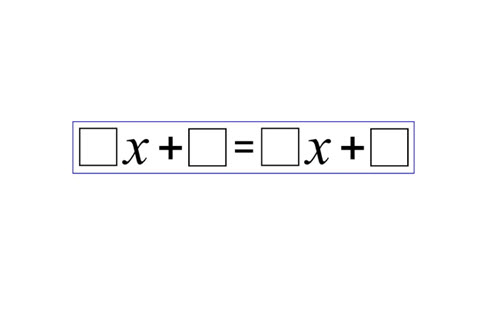Go to website

# Equal expressions

This lesson explores how well students can solve and create linear equations, determining whether each side of the equation is equal. The lesson involves students creating their own equations and trying to solve the value of x. Students work collaboratively in attempting to solve the equation, deciding if there is always a solution. This involves the process of establishing and utilising rules. The lesson is accompanied by downloadable resources for teacher use.

Year level(s) Year 8
Audience Teacher
Purpose Teaching resource, Content knowledge, Evidence-based approaches, Student task, Teaching strategies
Teaching strategies and pedagogical approaches Collaborative learning, Mathematics investigation, Questioning, Setting goals, Structuring lessons, Worked examples
Keywords unknown, rearrangement, equations, inductive, deductive, coeffecients

## Curriculum alignment

Curriculum connections Critical and creative thinking, Numeracy
Strand and focus Number, Algebra, Apply understanding, Build understanding
Topics Algebraic expressions, Data representation and interpretation, Linear relationships, Patterns and algebra
AC: Mathematics (V9.0) content descriptions
AC9M8A02
Graph linear relations on the Cartesian plane using digital tools where appropriate; solve linear equations and one-variable inequalities using graphical and algebraic techniques; verify solutions by substitution

Numeracy progression Number patterns and algebraic thinking (P7)